# Generating Spheroids, Ellipsoids, and Quadrics

## Getting Started

Here we explore the vtkQuadric method, which has been used to generate Figure 5-2 (§5.1, p. 77) of the VTK User's Guide, authored and published by Kitware, Inc., in an effort to learn how to draw two-dimensional spheroidal structures, three-dimensional ellipsoidal structures, and other analytically specifiable configurations.

1. Inside VisTrails, open, then execute "VisQuad.vt" without making any changes to the default pipeline or to any module parameters:

• The default workflow pipeline is shown in the top panel of the following figure. Upon execution, the default composite image that pops up in the VisTrails spreadsheet window is shown in the bottom panel.

## Various Modules

In the workflow image displayed above, the vtkQuadric module has been highlighted, so the inset window to the right of the workflow pipeline displays the values of the 10 separate model coefficients that have been used to generate the default "Quadric" configuration. Presumably, these values correspond, respectively, to coefficients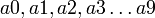$~a0, a1, a2, a3 \ldots a9$ as they appear in the quadric function definition as provided in the default module documentation and as reprinted here:

Superclass: vtkImplicitFunction

vtkQuadric evaluates the quadric function F(x,y,z) = a0*x^2 + a1*y^2 + a2*z^2 + a3*x*y + a4*y*z + a5*x*z + a6*x + a7*y + a8*z + a9. vtkQuadric is a concrete implementation of vtkImplicitFunction.

We conclude that the default image displayed above illustrates geometric properties of an underlying scalar field that has been generated from the function,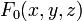$~F_0(x,y,z)$$~=$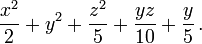$~\frac{x^2}{2} + y^2 + \frac{z^2}{5} + \frac{yz}{10} + \frac{y}{5}\, .$

### vtkSampleFunction

As implemented in this example visualization, this module assigns default values and behavior to all methods except one:

• SampleDimensions is specified by the three integers (30, 30, 30); this is presumably the number of (uniformly sized) grid cells in each of the three coordinate directions — respectively, (x, y, z) — that will be assigned values of the function,$~F_0(x, y, z)$.

While initially deciphering the behavior of this entire workflow, I wondered how the edges of the (black) bounding box were specified. Simultaneously, I wondered over what range of values of x, y, and z the grid resolution of (30, 30, 30) was being stretched. The answer to both of these questions is the same. The function is sampled over the range of coordinate values — and the (black) bounding box is drawn at the edges of this sampled region — as specified by the vtkSampleFunction method, ModelBounds.

• By experimentation, I discovered that the default coordinate values assigned to Modelbounds are, for x, y, and z, respectively, (-1.0, 1.0), (-1.0, 1.0), and (-1.0, 1.0). By assigning, instead, values of (0.0, 1.0), (0.0, 1.0), and (0.0, 1.0), precisely one octant of the original configuration is displayed.

### vtkContourFilter

As is explained at the top of p. 78 of the VTK User's Guide, five different contours — that is, five different surfaces differentiated from one another by color — have been drawn. The specific set of surfaces has been specified via the "GenerateValues" method inside the vtkContourFilter module. Clicking on (i.e., highlighting) the vtkContourFilter module in the VisTrails workflow reveals that three numbers have been specified:

• Two floating-point numbers identify the "scalar range" — in the default case, the specified range is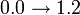$0.0 \rightarrow 1.2$;
• The single integer identifies the "number of contours to be generated in the range (end values inclusive).

We conclude that the five separately colored "surfaces" correspond to contours for which the function,$~F_0(x,y,z)$, is constant and has the following values:

 Contour Color: Associated Scalar Value: red yellow green light blue dark blue 0.0 0.3 0.6 0.9 1.2

### vtkPolyDataMapper

This default workflow contains two independent instances of the vtkPolyDataMapper module. The instance on the left plays a role in mapping the nested color contours to the image screen (that is, to the spreadsheet window) while the instance on the right plays a role in mapping the bounding box to the screen.

• vtkPolyDataMapper (left) — notice that a ScalarRange of (0.0, 1.2) has been explicitly assigned, which is exactly the same "scalar range" that has been explicitly assigned inside the vtkContourFilter module. This means that the default color table, which spans from red to dark blue, should be stretched to cover the entire range of scalar values that is being probed. The colors of the five distinct contours can be changed by specifying a different ScalarRange inside this instance of vtkPolyDataMapper (left). For example, setting ScalarRange = (0.0, 0.6) will squeeze the full dynamic range of the color table into a narrower range of scalar values; the first three contours —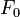$~F_0$ = 0.0, 0.3, and 0.6 — will be colored red, green, and dark blue, while the last two contours —$~F_0$ = 0.9 and 1.2 — will be pegged to the color dark blue because they are outside of the new identified range.
• vtkPolyDataMapper (right) — notice that default values and behaviors are accepted throughout.

We should point out that, in this default workflow, the color of the bounding box is set (to black) by the Color method inside of the vtkProperty module; this can be readily changed to generate a bounding box whose edges are a different color, for example, red.

## Playing With Parameters

My desire is to generate a set of 10 concentric ellipsoidal surfaces having semi-axes of length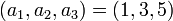$~(a_1, a_2, a_3) = (1, 3, 5)$ using the default workflow discussed above. In order to accomplish this and then display an interesting cut-away projection of the configuration, I have changed the (above) default parameter settings to the ones shown in the following table. Upon execution, the resulting image that was displayed in the VisTrails spreadsheet window is presented in the panel to the right of the table.

Parameter Values

Module

Method/Parameter

Assigned Value(s)

Coefficients

(1, 3, 5, 0, 0, 0, 0, 0, 0, 0)

vtkSampleFunction:

ModelBounds

(0.0, 1.0), (-1.0, 1.0), (0.0, 1.0)

SampleDimensions

(40, 40, 40)

vtkContourFilter:

GenerateValues

(10, 0.05, 0.9)

vtkPolyDataMapper (left):

ScalarRange

(0.0, 0.9)

vtkActor (both left & right):

RotateX

-180

RotateY

-45

## Animation of Spinning Ellipsoid

Next, I wanted to construct an animation sequence of an ellipsoid that is spinning about its shortest axis. I also wanted to attach three colored "vectors" that are permanently aligned with the three principal axes of the ellipsoid; I did not think this would be difficult because, in connection with the Simple Cube Tutorial, I had already developed a workflow that generates three such coordinate-identifying vectors. [As shown in the workflow, below, the linear "stem" of each coordinate-axis vector was constructed as a long, thin cylinder using vtkCylinderSource, and the crowning "arrowhead" of each was constructed using vtkConeSource.]

My initial thought was that I could generate the desired "spin" by simply "rolling" the camera. More specifically, I thought I could use the parameter exploration feature in VisTrails to vary the "Roll" angle inside of the vtkCamera module. I quickly found out that this approach would work only if I pointed the camera down the z-axis. It would not work if I wanted to view the spinning ellipsoid from some other "azimuthal" angle, because then the vtkCamera's "Roll" axis does not coincide with the desired "spin" axis of the ellipsoid.

After some thought, I realized that I needed, instead, to vary the "RotateZ" parameter inside of the vtkActor module … well, actually, this parameter would need to simultaneously be varied in all of the vtkActor modules. The VisQuad.vt workflow discussed above has two Actors — one handling the ellipsoidal contours and the other handling the bounding box — and an additional six Actors would be needed if I was going to simultaneously spin the coordinate-axis vectors. And the desire to spin those vectors posed a problem because, in the workflow that I originally constructed in order to build the vectors, I had already assigned values to the "RotateZ" parameter inside two vtkActor modules in order to properly align the "arrowhead" with the "stem" of two vectors.

I accomplished all the desired tasks using the workflow shown immediately above, in the lefthand panel of Figure 2; the resulting animation is displayed to the right of the workflow image. Here are a couple of the key steps that were taken:

• The vtkActors being used to generate the three coordinate-axis vectors were released from the responsibility of aligning the "arrowhead" with the "stem." To accomplish this, I inserted an additional pair of modules — one vtkTransform and one vtkTransformPolyDataFilter — into the subsets of the workflow (see the blue-bordered boxes in the Figure 2 workflow) that generates both the X-axis vector and the Y-axis vector. In both cases, the arrowhead-stem alignment is accomplished via this additional transformation step.
• A "Float" module — named "SpinAngle" (see the Figure 2 workflow) — was added to the workflow to serve as the mechanism by which the ellipsoid's spin-angle is specified. The value of this floating-point variable is fed into all of the vtkActor modules and, in each, is identified as the assigned value of "RotateZ"; this was accomplished by adding an additional input port to each vtkActor module.
• In the end, I decided not to feed the SpinAngle parameter value into the vtkActor module (a) that assists in defining the Z-coordinate axis vector, or (b) that assists in defining the bounding box. Because the ellipsoid is being spun about the Z-axis, the orientation of that axis is necessarily independent of SpinAngle; and, I thought that the animation looked better without spinning the bounding box.